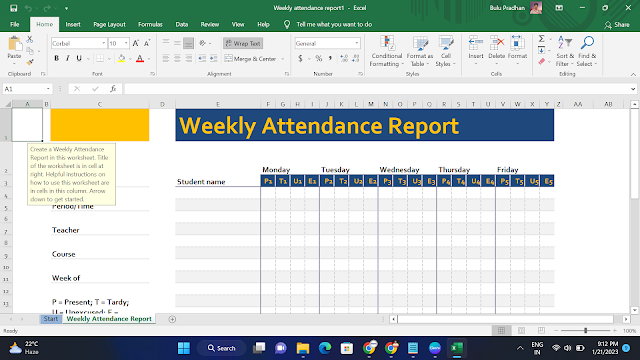# Most Popular Excel Formulas and With Explain

Excel has a wide range of formulas that can be used to perform various calculations and operations on data. Here are some of the most commonly used formulas in Excel, along with a brief explanation of what they do:Most Popular Excel Formulas

• SUM: This formula adds up the values in a range of cells. For example, =SUM(A1:A5) would add up the values in cells A1 through A5.
• AVERAGE: This formula calculates the average of a range of cells. For example, =AVERAGE(A1:A5) would give the average of values in cells A1 through A5.
• COUNT: This formula counts the number of cells in a range that contain numbers. For example, =COUNT(A1:A5) would count the number of cells in the range A1 through A5 that contain numbers.
• MIN: This formula returns the smallest value in a range of cells. For example, =MIN(A1:A5) would give the smallest value in cells A1 through A5.
• MAX: This formula returns the largest value in a range of cells. For example, =MAX(A1:A5) would give the largest value in cells A1 through A5.
• IF: This formula tests a condition and returns one value if the condition is true and another value if the condition is false. For example, =IF(A1>5, "Greater than 5", "Less than or equal to 5") would return "Greater than 5" if the value in cell A1 is greater than 5, and "Less than or equal to 5" if the value in cell A1 is less than or equal to 5.
• VLOOKUP: This formula searches for a value in the leftmost column of a table and returns a value from a specified column in the same row. For example, =VLOOKUP(A1,A2:C5,3,FALSE) would search for the value in cell A1 in the leftmost column of the table in range A2:C5 and return the value from the third column in the same row.
• INDEX: This formula returns a value from a specified cell in a range or array. For example, =INDEX(A1:C3,2,3) would return the value in the cell at the intersection of the second row and third column of the range A1:C3.
• CONCATENATE: This formula combines text from two or more cells into one cell. For example, =CONCATENATE(A1,B1) would combine the text in cells A1 and B1 into one cell.

These are just a few examples of the many formulas available in Excel. Each one has its own specific use case and can be useful in different situations.

1.2.